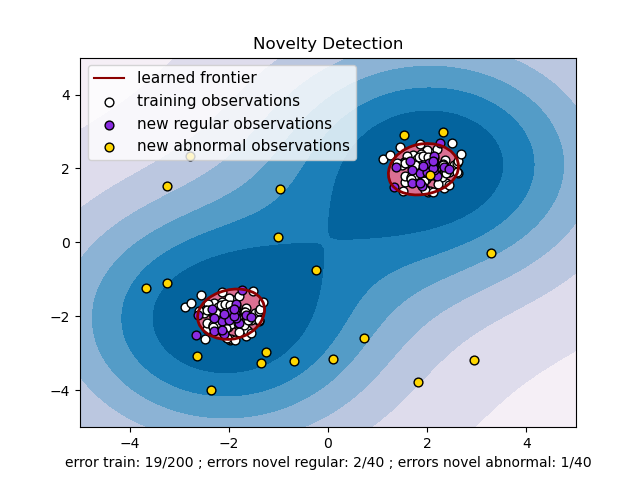# One-class SVM with non-linear kernel (RBF)¶

An example using a one-class SVM for novelty detection.

One-class SVM is an unsupervised algorithm that learns a decision function for novelty detection: classifying new data as similar or different to the training set./home/circleci/project/examples/svm/plot_oneclass.py:57: MatplotlibDeprecationWarning:

The collections attribute was deprecated in Matplotlib 3.8 and will be removed two minor releases later.


import matplotlib.font_manager
import matplotlib.pyplot as plt
import numpy as np

from sklearn import svm

xx, yy = np.meshgrid(np.linspace(-5, 5, 500), np.linspace(-5, 5, 500))
# Generate train data
X = 0.3 * np.random.randn(100, 2)
X_train = np.r_[X + 2, X - 2]
# Generate some regular novel observations
X = 0.3 * np.random.randn(20, 2)
X_test = np.r_[X + 2, X - 2]
# Generate some abnormal novel observations
X_outliers = np.random.uniform(low=-4, high=4, size=(20, 2))

# fit the model
clf = svm.OneClassSVM(nu=0.1, kernel="rbf", gamma=0.1)
clf.fit(X_train)
y_pred_train = clf.predict(X_train)
y_pred_test = clf.predict(X_test)
y_pred_outliers = clf.predict(X_outliers)
n_error_train = y_pred_train[y_pred_train == -1].size
n_error_test = y_pred_test[y_pred_test == -1].size
n_error_outliers = y_pred_outliers[y_pred_outliers == 1].size

# plot the line, the points, and the nearest vectors to the plane
Z = clf.decision_function(np.c_[xx.ravel(), yy.ravel()])
Z = Z.reshape(xx.shape)

plt.title("Novelty Detection")
plt.contourf(xx, yy, Z, levels=np.linspace(Z.min(), 0, 7), cmap=plt.cm.PuBu)
a = plt.contour(xx, yy, Z, levels=, linewidths=2, colors="darkred")
plt.contourf(xx, yy, Z, levels=[0, Z.max()], colors="palevioletred")

s = 40
b1 = plt.scatter(X_train[:, 0], X_train[:, 1], c="white", s=s, edgecolors="k")
b2 = plt.scatter(X_test[:, 0], X_test[:, 1], c="blueviolet", s=s, edgecolors="k")
c = plt.scatter(X_outliers[:, 0], X_outliers[:, 1], c="gold", s=s, edgecolors="k")
plt.axis("tight")
plt.xlim((-5, 5))
plt.ylim((-5, 5))
plt.legend(
[a.collections, b1, b2, c],
[
"learned frontier",
"training observations",
"new regular observations",
"new abnormal observations",
],
loc="upper left",
prop=matplotlib.font_manager.FontProperties(size=11),
)
plt.xlabel(
"error train: %d/200 ; errors novel regular: %d/40 ; errors novel abnormal: %d/40"
% (n_error_train, n_error_test, n_error_outliers)
)
plt.show()


Total running time of the script: (0 minutes 0.425 seconds)

Gallery generated by Sphinx-Gallery